Two physical pendulums perform small oscillations about the same horizontal axis with frequencies w1 and w2. Their moments of inertia relative to the given axis are equal to I1 and I2 respectively. In a state of stable equilibrium, the pendulums were fastened rigidly together. What will be the frequency of small oscillations of the compound pendulum?

Anonymous User Physics Oscillation 02 May, 2020 85 views

A physical pendulum performs small oscillations about the horizontal axis with frequency co = 15.0 s-1 . When a small body of mass m = 50 g is fixed to the pendulum at a distance l = 20 cm below the axis, the oscillation frequency becomes equal to w2 = 10.0 s-1. Find the moment• of inertia of the pendulum relative to the oscillation axis.

Anonymous User Physics Oscillation 02 May, 2020 61 views

A physical pendulum is positioned so that its centre of gravity
is above the suspension point. From that position the pendulum
started moving toward the stable equilibrium and passed it with an
angular velocity w. Neglecting the friction find the period of small
oscillations of the pendulum.

Anonymous User Physics Oscillation 02 May, 2020 63 views

An arrangement illustrated in Fig consists of a horizontal uniform disc D of mass m and radius R and a thin rod AO whose torsional coefficient is equal to k. Find the amplitude and the energy of small torsional oscillations if at the initial moment the disc was deviated through an angle φ, from the equilibrium position and then imparted an angular velocity φo.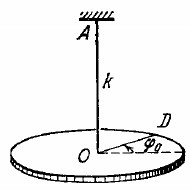Anonymous User Physics Oscillation 02 May, 2020 65 viewsA uniform rod of mass m = 1.5 kg suspended by two identical threads 1 = 90 cm in length (Fig) was turned through a small angle about the vertical axis passing through its middle point C. The threads deviated in the process through an angle a = 5.0°.Then the rod was released to start performing small oscillations.Find:
(a) the oscillation period;
(b) the rod's oscillation energy.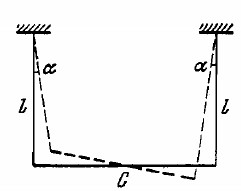Anonymous User Physics Oscillation 02 May, 2020 57 views

A pendulum is constructed as a light thin-walled sphere of radius R filled up with water and suspended at the point 0 from a light rigid rod (Fig). The distance between the point 0 and the centre of the sphere is equal to 1. How many times will the small oscillations of such a pendulum change after the water freezes? The viscosity of water and the change of its volume on freezing are to be neglected.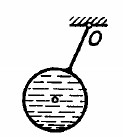Anonymous User Physics Oscillation 02 May, 2020 76 views

A particle of mass m moves in the plane xy due to the force varying with velocity as F = a (yi — xj), where a is a positive constant,i and j are the unit vectors of the x and y axes. At the initial moment t = 0 the particle was located at the point x = y = 0 and possessed a velocity v0 directed along the unit vector j. Find the law of motion x (t) , y (t) of the particle, and also the equation of its trajectory.

Anonymous User Physics Oscillation 02 May, 2020 98 views

A body of mass m fell from a height h onto the pan of a spring balance (Fig.)The masses of the pan and the spring are negligible, the stiffness of the latter is x. Having stuck to the pan, the body starts performing harmonic oscillations in the vertical direction. Find the amplitude and the energy of these oscillations.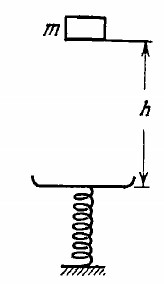Anonymous User Physics Oscillation 02 May, 2020 63 views

A particle of mass in moves due to the force F = - αmr,where a is a positive constant, r is the radius vector of the particle relative to the origin of coordinates. Find the trajectory of its motion if at the initial moment r = roi and the velocity v = voj, where I and j are the unit vectors of the x and y axes.

Anonymous User Physics Oscillation 02 May, 2020 57 views

A mathematical pendulum oscillates in a medium for which the logarithmic damping decrement is equal to 20 = 1.50. What will be the logarithmic damping decrement if the resistance of the medium increases n = 2.00 times? How many times has the resistance of the medium to be increased for the oscillations to become impossible?

Anonymous User Physics Oscillation 02 May, 2020 55 views

A body of mass in was suspended by a non-stretched spring, and then set free without push. The stiffness of the spring is x.Neglecting the mass of the spring, find:
(a) the law of motion y (t) , where y is the displacement of the body from the equilibrium position;
(b) the maximum and minimum tensions of the spring in the process of motion.

Anonymous User Physics Oscillation 02 May, 2020 61 views

A plank with a body of mass m placed on it starts moving straight up according to the law y = a (1 — cos wt), where y is the displacement from the initial position w = 11 /s. Find:
(a) the time dependence of the force that the body exerts on the plank if a = 4.0 cm; plot this dependence;
(b) the minimum amplitude of oscillation of the plank at which the body starts falling behind the plank;
(c) the amplitude of oscillation of the plank at which the body springs up to a height h = 50 cm relative to the initial position (at the moment t = 0).

Anonymous User Physics Oscillation 02 May, 2020 84 views

Find the time dependence of the angle of deviation of a mathematical pendulum 80 cm in length if at the initial moment the pendulum (a) was  deviated through the angle 3.0° and then set free without push;

Anonymous User Physics Oscillation 02 May, 2020 59 views

A plank with a bar placed on it performs horizontal harmonic oscillations with amplitude a = 10 cm. Find the coefficient of friction between the bar and the plank if the former starts sliding along the plank when the amplitude of  scillation of the plank becomes less than T = 1.0 s.

Anonymous User Physics Oscillation 02 May, 2020 86 views

In the arrangement shown in Fig the sleeve M of mass m=0.20 kg is fixed between two identical springs whose combined stiffness is equal to x = 20 N/m. The sleeve can slide without friction over a horizontal bar AB. The arrangement rotates with a constant angular velocity w = 4.4 rad/s about a vertical axis passing through the middle of the bar. Find the period of small oscillations of the sleeve. At what values of o will there be no oscillations of the sleeve?.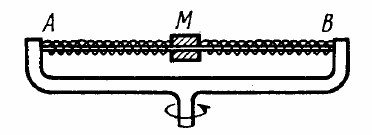Anonymous User Physics Oscillation 02 May, 2020 67 views

Imagine a shaft going all the way through the Earth from pole to pole along its rotation axis. Assuming the Earth to be a homogeneous ball and neglecting the air drag, find:

(a) the equation of motion of a body falling down into the shaft;

(b) how long does it take the body to reach the other end of the shaft;

(c) the velocity of the body at the Earth's centre

Anonymous User Physics Oscillation 02 May, 2020 68 views

A uniform rod is placed on two spinning wheels as shown in Fig. The axes of the wheels are separated by a distance 1= 20 cm,the coefficient of friction between the rod and the wheels is k = 0.18. Demonstrate that in this case the rod performs harmonic oscillations. Find the period of these oscillations.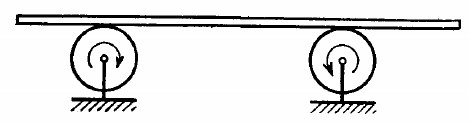Anonymous User Physics Oscillation 02 May, 2020 111 views

Determine the period of oscillations of mercury of mass = 200 g poured into a bent tube (Fig.) whose right arm forms an angle 0 = 30° with the vertical. The cross-sectional area of the tube is S = 0.50 cm2. The viscosity of mercury is to be neglected.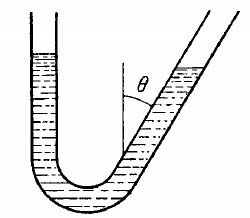Anonymous User Physics Oscillation 02 May, 2020 77 views

A small body of mass in is fixed to the middle of a stretched string of length 2l. In the equilibrium position the string tension is equal to To. Find the angular frequency of small oscillations of the body in the transverse direction. The mass of the string is negligible,the gravitational field is absent.

Anonymous User Physics Oscillation 02 May, 2020 63 views

Find the period of small vertical oscillations of a body with mass m in the system illustrated in Fig.The stiffness values of the springs are xi and x2, their masses are negligible.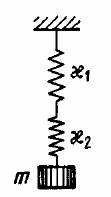Anonymous User Physics Oscillation 02 May, 2020 65 views

Determine the period of small longitudinal oscillations of a body with mass m in the system shown in Fig.  The stiffness values of the springs are xi and x2. The friction and the masses of the springs are negligible.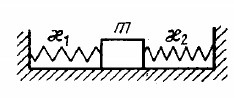Anonymous User Physics Oscillation 02 May, 2020 57 views

Calculate the period of small oscillations of a hydrometer (Fig) which was slightly pushed down in the vertical direction.The mass of the hydrometer is m = 50 g, the radius of its tube is r = 3.2 mm, the density of the liquid is p = 1.00 g/cm3. The resistance of the liquid is assumed to be negligible.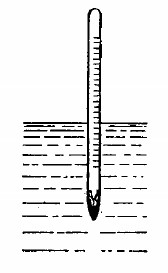Anonymous User Physics Oscillation 02 May, 2020 62 views

ball is suspended by a thread of length l at the point 0 on
the wall, forming a small angle a with the vertical (Fig. 4.1). Then
Fig. 4.1, Fig. 4.2.
the thread with the ball was deviated through a small angle 13(l > a)
and set free. Assuming the collision of the ball against the wall to
be perfectly elastic, find the oscillation period of such a pendulum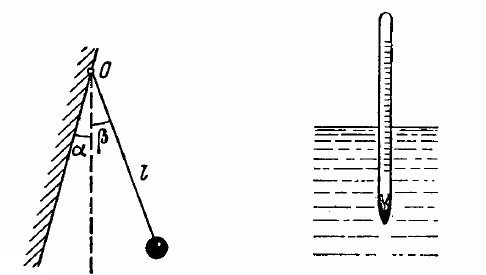Anonymous User Physics Oscillation 01 May, 2020 84 views

Determine the period of small oscillations of a mathematical pendulum, that is a ball suspended by a thread 1 = 20 cm in length,if it is located in a liquid whose density is = 3.0 times less than that of the ball. The resistance of the liquid is to be neglected.

Anonymous User Physics Oscillation 01 May, 2020 65 views

Find the period of small oscillations in a vertical plane performed by a ball of mass m = 40 g fixed at the middle of a horizontally stretched string 1 = 1.0 m in length. The tension of the string is assumed to be constant and equal to F = 10 N.

Anonymous User Physics Oscillation 01 May, 2020 81 views

A particle of mass m is located in a unidimensional potential field where the potential energy of the particle depends on the coordinate x as U (x) = U0 (1 — cos ax); U0 and a are constants. Find the period of small oscillations that the particle performs about the equilibrium position.

Anonymous User Physics Oscillation 01 May, 2020 71 views

Find the trajectory equation y (x) of a point if it moves according
to the following laws:
(a) x = a sin wt, y = a sin 2wt;
(b) x = a sin wt, y = a cos 2wt.
Plot these trajectories.

Anonymous User Physics Oscillation 01 May, 2020 98 views

A point moves in the plane xy according to the law x = a sin wt   y = b cos wt, where a, b, and w are positive constants. Find:
(a) the trajectory equation y (x) of the point and the direction of its motion along this trajectory;
(b) the acceleration w of the point as a function of its radius vector r relative to the origin of coordinates.

Anonymous User Physics Oscillation 01 May, 2020 98 views

A point A oscillates according to a certain harmonic law in the reference frame K' which in its turn performs harmonic oscillations relative to the reference frame K. Both oscillations occur along the same direction. When the K' frame oscillates at the frequency 20 or 24 Hz, the beat frequency of the point A in the K frame turns out to be equal to v. At what frequency of oscillation of the frame K' will the beat frequency of the point A become equal to 2v?

Anonymous User Physics Oscillation 01 May, 2020 68 views

A point A oscillates according to a certain harmonic law in the reference frame K' which in its turn performs harmonic oscillations relative to the reference frame K. Both oscillations occur along the same direction. When the K' frame oscillates at the frequency 20 or 24 Hz, the beat frequency of the point A in the K frame turns

Anonymous User Physics Oscillation 01 May, 2020 65 views

## When 1 mole of PbS reacts completely withWhen 1 mole of PbS reacts completely with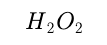Anonymous User Chemistry Hydrogen 01 May, 2020 62 views

The superposition of two harmonic oscillations of the same direction results in the oscillation of a point according to the law x = a cos 2.1t cos 50.0t, where t is expressed in seconds. Find the angular frequencies of the constituent oscillations and the period with which they beat.

Anonymous User Physics Oscillation 01 May, 2020 58 views

Using graphical means, find an amplitude a of oscillations resulting from the superposition of the following oscillations of the same direction:
(a) x1 =3 .0 cos (wt -1- π/3), x 2 =8 .0sin (wt π/6);

Anonymous User Physics Oscillation 01 May, 2020 58 views

For a first order reaction A -> P, the temperature (T) dependent rate constant (k) was found to follow the equation
log k = – (2000) 1/T + 6.0. The pre-exponential factor A and the activation energy Ea, respectively, are

Anonymous User Chemistry Chemical Kinetics 01 May, 2020 71 views

For the elementary reaction M -> N, the rate of disappearance of M increases by a factor of 8 upon doubling the concentration of M. The order of the reaction with respect to M is

Anonymous User Chemistry Chemical Kinetics 01 May, 2020 102 views

Consider a reaction aG + bH -> Products. When concentration of both the reactants G and H is doubled, the rate increases by eight times. However, when concentration of G is doubled keeping the concentration of H fixed, the rate is doubled. The overall order of the reaction is

Anonymous User Chemistry Chemical Kinetics 01 May, 2020 67 views

A positron is emitted from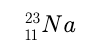. The ratio of the atomic mass and atomic number of the resulting nuclide is

Anonymous User Chemistry Chemical Kinetics 01 May, 2020 62 views